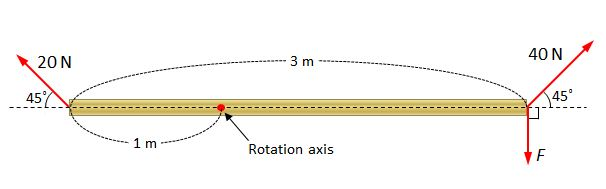# TorqueThe above shows a $3$ m rod fixed by the rotation axis at the point where the internal division ratio is $1:2.$ If three forces indicated by the three red arrows--$20$ N, $40$ N, F--are acting and the rod does not rotate, what is the magnitude of $F$?

×